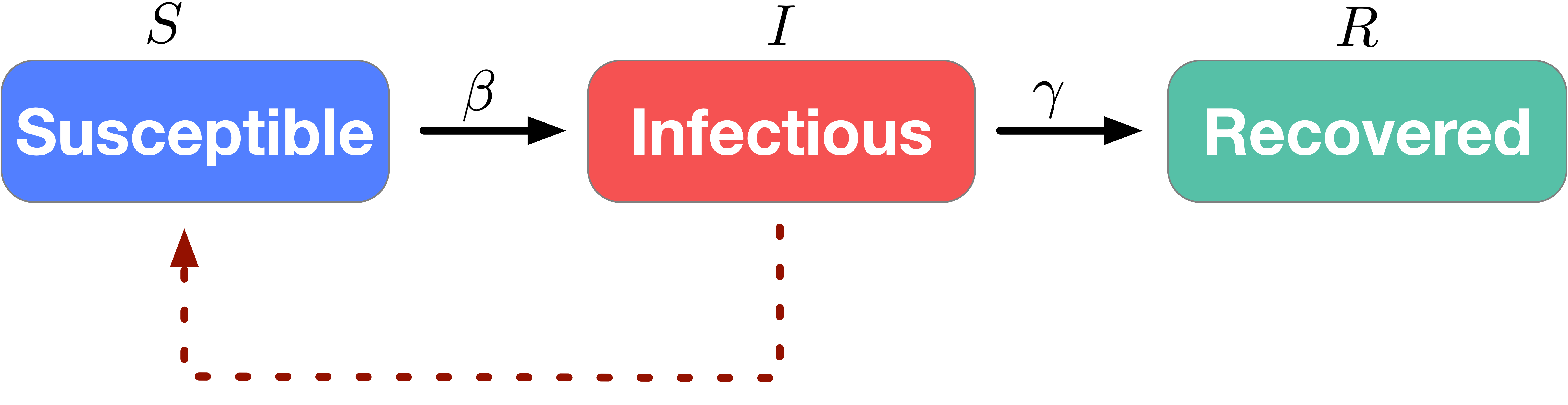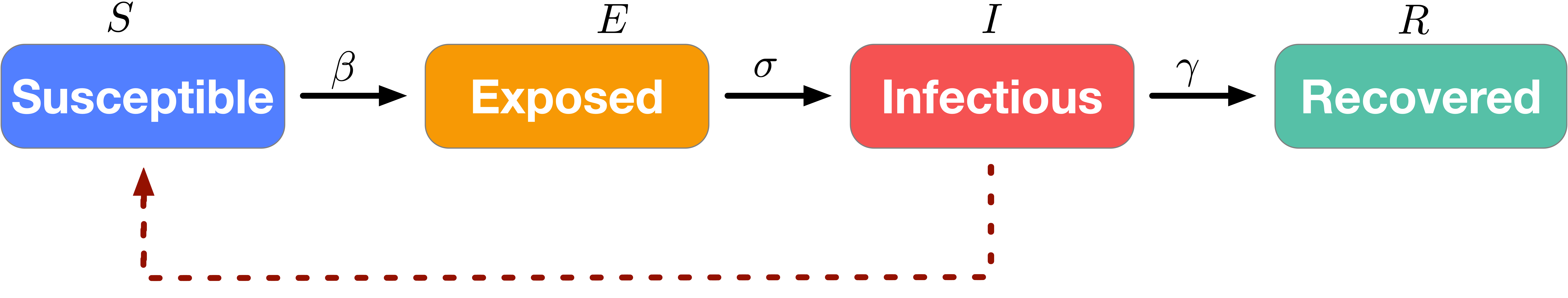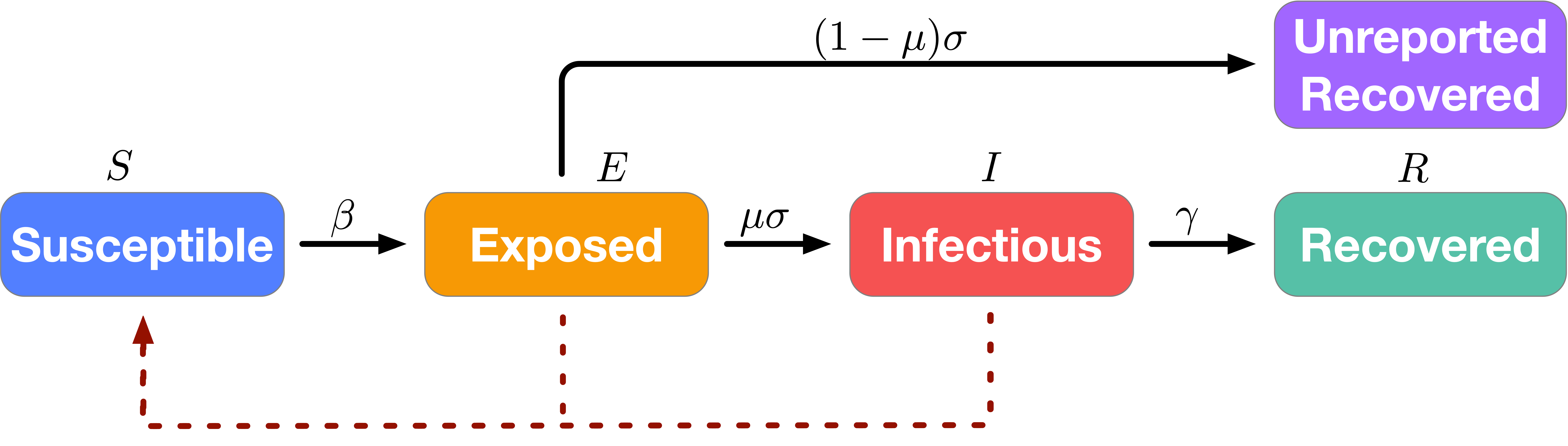We proposed an improved SEIR model for predicting the dynamics among the cumulative confirmed cases and death of COVID-19. Before we introduce this model, we start with some basic epidemic models.

#### The SIR Model

SIR1 is an epidemic model that shows the change of infection rate over time. As illustrated in Figure 1, the SIR model characterizes the dynamic interplay among the susceptible individuals (S), infectious individuals (I) and recovered/deceased individuals (R) in a certain place. In the SIR model, the susceptible individuals may become infectious individuals over time, which depends on the spread rate of the virus, often called the contact rate. Recovered individuals are assumed to be immune to the virus and thus cannot become susceptible again.Figure 1. Illustration of the SIR model.

To characterize this dynamics, let's define parameters at time $t$ as follows:

• $S_t$: the number of susceptible individuals
• $I_t$: the number of infectious individuals
• $R_t$: the number of recovered/deceased/immune individuals
To simplify the analysis, we assume for now that the total population in the certain area is fixed as $N$. The evolving equations of the above parameters over time are defined as follows: \begin{align} \frac{d S_t}{d t}&= -\frac{\beta I_t S_t}{N}\\ \frac{d I_t}{d t}&= \frac{\beta I_t S_t}{N} - \gamma I_t\\ \frac{d R_t}{d t}&=\gamma I_t \end{align} where $\beta$ is the contact rate between the Susceptible and Infectious groups, and $\gamma$ is the transition rate between the Infectious and Recovered groups. The above ordinary differential equations indicate that at every time unit the total number of susceptible individuals will decrease by a quantity $-\beta I_t S_t/N$, who will transit into the infectious group. Apart from the increase from the transition of susceptible individuals, the size of the infectious group will also decrease by a factor of $\gamma$. In the COVID-19 case, the infection ratio $\beta$ could be scaled with $1/S_t$ since the population is not fully mixed and people are quarantined at home.

#### Incubation Period: The SEIR Model

For other diseases, there is often an incubation period during which individuals who have been exposed to the virus may not be as contagious as the infectious individuals. Therefore, it is important to separately model these cases as the "Exposed" group. As is shown in the following figure, this mode is usually referred to as SEIR2.Figure 2. Illustration of the SEIR model.

The dynamics of SEIR introduces a new compartment $E_t$, which models the number of individuals that are exposed to coronavirus but have not developed obvious symptoms. Among all the exposed cases, there are only a fraction $\sigma$ of people who will develop observable symptoms in a time unit.

\begin{align} \frac{d S_t}{d t}&= -\frac{\beta I_t S_t}{N}\\ \frac{d E_t}{d t}&= \frac{\beta I_t S_t}{N} -\sigma E_t\\ \frac{d I_t}{d t}&= \sigma E_t - \gamma I_t\\ \frac{d R_t}{d t}&=\gamma I_t \end{align}

Compared with the SIR model, SEIR has more elaborated model parameters. The parameters $\sigma,\beta$ and $\gamma$ can be learned from the reported data.

#### Unreported Recovery: The SuEIR Model

It is observed that COVID-19 has an incubation period ranging from 2 to 14 days3. However, during this period, individuals who have been exposed to the virus can also infect the susceptible group. In practice, the common situation is that the number of reported cases (including confirmed cases and recovered cases) are not equal to their real numbers as many infectious cases have not been tested, which will not pass to the next compartment. Therefore, we use the similar idea of SEIR and proposed a new epidemic model that takes the untested/unreported cases into consideration, which are illustrated in Figure 3.Figure 3. Illustration of the SuEIR model.

In particular, the compartment Exposed in our model is considered as the cases that have already been infected and have not been tested. Therefore, they also have the capability to infect the susceptible individuals. Moreover, some of such cases can receive a test and be passed to the Infectious compartment (as well as reported to the public), while the rest of them will recover/die but not appear in the publicly reported cases. Therefore, we introduce a new parameter $\mu<1$ in the evolution dynamics of $I_t$ to control the ratio of the exposed cases that are confirmed and reported to the public.

\begin{align*} \frac{d S_t}{d t}&= -\frac{\beta (I_t+E_t) S_t}{N}\\ \frac{d E_t}{d t}&= \frac{\beta (I_t+E_t) S_t}{N} -\sigma E_t\\ \frac{d I_t}{d t}&= \mu \sigma E_t - \gamma I_t\\ \frac{d R_t}{d t}&=\gamma I_t \end{align*}

#### Training the SuEIR Model Using Machine Learning Methods

In order to find the optimal parameters of the SuEIR model, defined by $\boldsymbol{\theta} = (\beta, \sigma, \gamma, \mu)$, we apply gradient-based optimizers to minimizing the following loss function \begin{align*} L(\boldsymbol{\theta}; \mathbf {I }, \mathbf {R}) = \frac{1}{T}\sum_{t=1}^T (\hat I_t - I_t)^2 + \lambda (\hat R_t - R_t)^2, \end{align*} where $\hat I_t$ and $\hat R_t$ denote the reported numbers of confirmed cases and recover cases at time $t$ (or date $t$), $I_t$ and $R_t$ denote the numbers of confirmed cases and recover cases computed based on our model, and $\lambda>0$ is a tuning parameter in order to balance the importance between the prediction error in terms of the confirmed cases and recover cases. In particular, since the true model is defined by ordinary differential equations (ODEs), in our experiment we apply numerical ODE solvers to compute $S_t$ and $R_t$ given the model parameters $\boldsymbol{\theta}$ and initial quantities $S_0$, $E_0$, $I_0$, and $R_0$.

In terms of the initialization, we can directly set $I_0 = \hat I_0$ and $R_0 = \hat R_0$. Additionally, one can typically set $S_0+E_0+I_0+R_0 = N$, where $N$ is the total population of the region (which can be either a country or a state/province). However, since most of the states in the US have already issued the safer-at-home rule, the actual total number of cases in the SuEIR model, denoted by $N_0$, must be less than $N$. Moreover, we also need to point out that the initialization of $E$, i.e., $E_0$, is a bit tricky since we do not know the number of infected cases before testing them. However, it is also not reasonable to set $E_0=0$ since generally there have already existed a large number of infected cases when the governments begin to perform the test and report. In our experiment, we train multiple models with different choices of $N_0$ and $E_0$ and select ones with reasonable training loss.

#### Prediction of Confirmed Cases in California

Figure 4 shows the prediction of our SuEIR model for the confirmed cases in California. Baseline models are SIR, Arima4, and Gaussian error fit5 (i.e., fitted by Gaussian error function). All the models are trained based on the actual numbers up to 04/03/2020. The results show that the increase of confirmed cases will slow down around the mid of May, and the projected confirmed cases in California is around 51000.

Figure 4. Prediction of cumulative confirmed cases by SuEIR model.
Similarly, we plot the predicted cumulative death cases in California in Figure 5. The results show that the increase of deaths will slow down around the beginning of June, and the projected deaths in California is around 2100.

Figure 5. Prediction of cumulative death cases by SuEIR model.